# 1. Implement a Rational Number class with the following specifications.Data membersa) numerator and denominatorFunctionsa) Constructors:1) default constructor2) single parameter constructor to create numerator/13) dual parameter constructor to create numerator/denominator4) Use constructor delegation across all constructors.b) Accessors and Mutators for both data members.c) Static recursive GCD function using Euclid's algorithm.d) Static LCM function for two numbers.e) Reduce function simplify a rational number.This function modifies its calling object.f) Your program should work with the supplied driver program.NotesLCM (Least Common Multiple)This function returns the smallest multiple of a and b.Step 1: Multiply a and b to find a common multiple.Step 2: Divide the common multiple by the GCD of a and b.Step 3: Return the result of Step 2.Reduce:This function reduces a fraction to simplest terms (i.e. 9/12 to 3/4).Step 1: Find the GCD of the numerator and denominator.Step 2: Divide the numerator by GCD and store as the new numerator.Step 3: Divide the denominator by GCD and store as the new denominator.Static FunctionsRecall that static functions are class functions and not associated withinstances of the class (objects). In this class, the static functions GCDand LCM should accept inputs any input pair (a and b) and return an answerbased upon that input pair. As such, these functions can be used by theprogrammer upon Rational Number objects or random values for a and b. Example Driver Programint main() {cout

Question
29 views

I'm stuck on this question and I don't know how I should be approaching this. What should I do?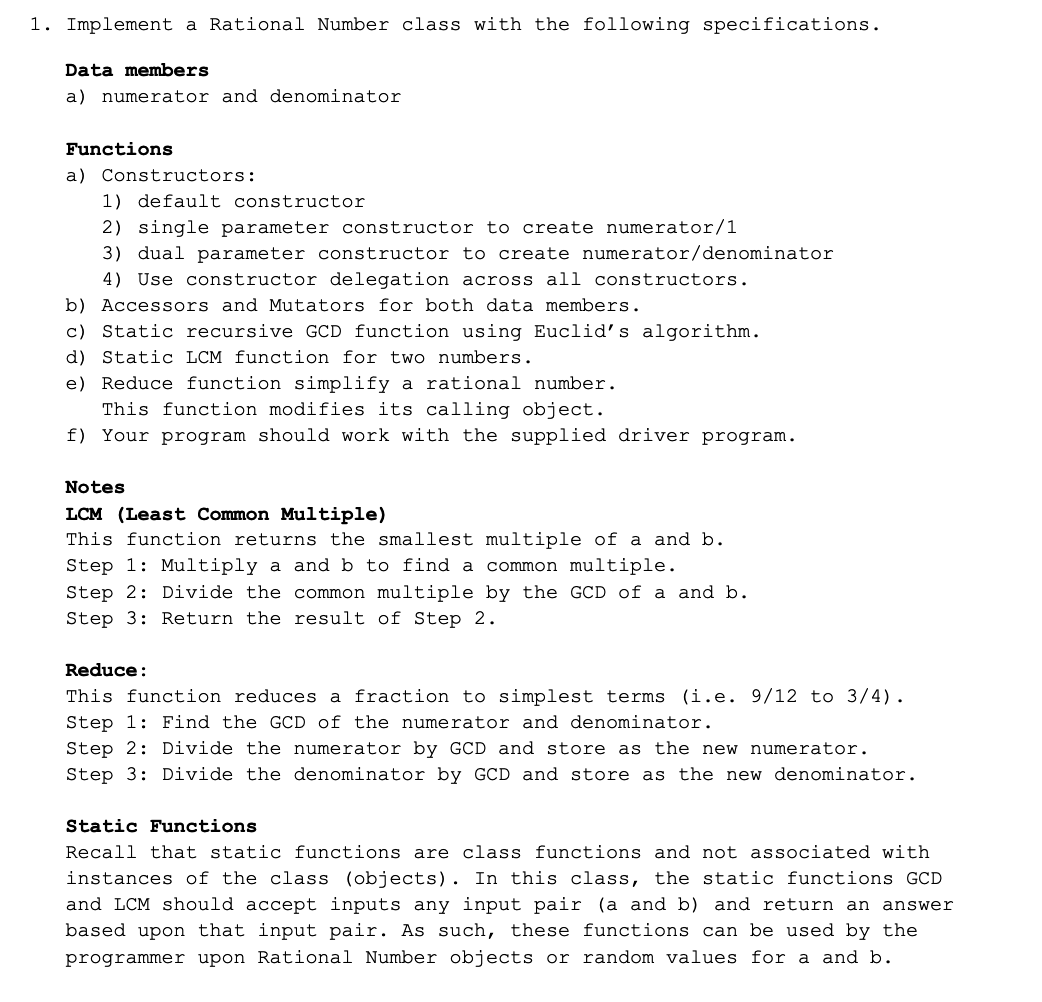help_outlineImage Transcriptionclose1. Implement a Rational Number class with the following specifications. Data members a) numerator and denominator Functions a) Constructors: 1) default constructor 2) single parameter constructor to create numerator/1 3) dual parameter constructor to create numerator/denominator 4) Use constructor delegation across all constructors. b) Accessors and Mutators for both data members. c) Static recursive GCD function using Euclid's algorithm. d) Static LCM function for two numbers. e) Reduce function simplify a rational number. This function modifies its calling object. f) Your program should work with the supplied driver program. Notes LCM (Least Common Multiple) This function returns the smallest multiple of a and b. Step 1: Multiply a and b to find a common multiple. Step 2: Divide the common multiple by the GCD of a and b. Step 3: Return the result of Step 2. Reduce: This function reduces a fraction to simplest terms (i.e. 9/12 to 3/4). Step 1: Find the GCD of the numerator and denominator. Step 2: Divide the numerator by GCD and store as the new numerator. Step 3: Divide the denominator by GCD and store as the new denominator. Static Functions Recall that static functions are class functions and not associated with instances of the class (objects). In this class, the static functions GCD and LCM should accept inputs any input pair (a and b) and return an answer based upon that input pair. As such, these functions can be used by the programmer upon Rational Number objects or random values for a and b. fullscreen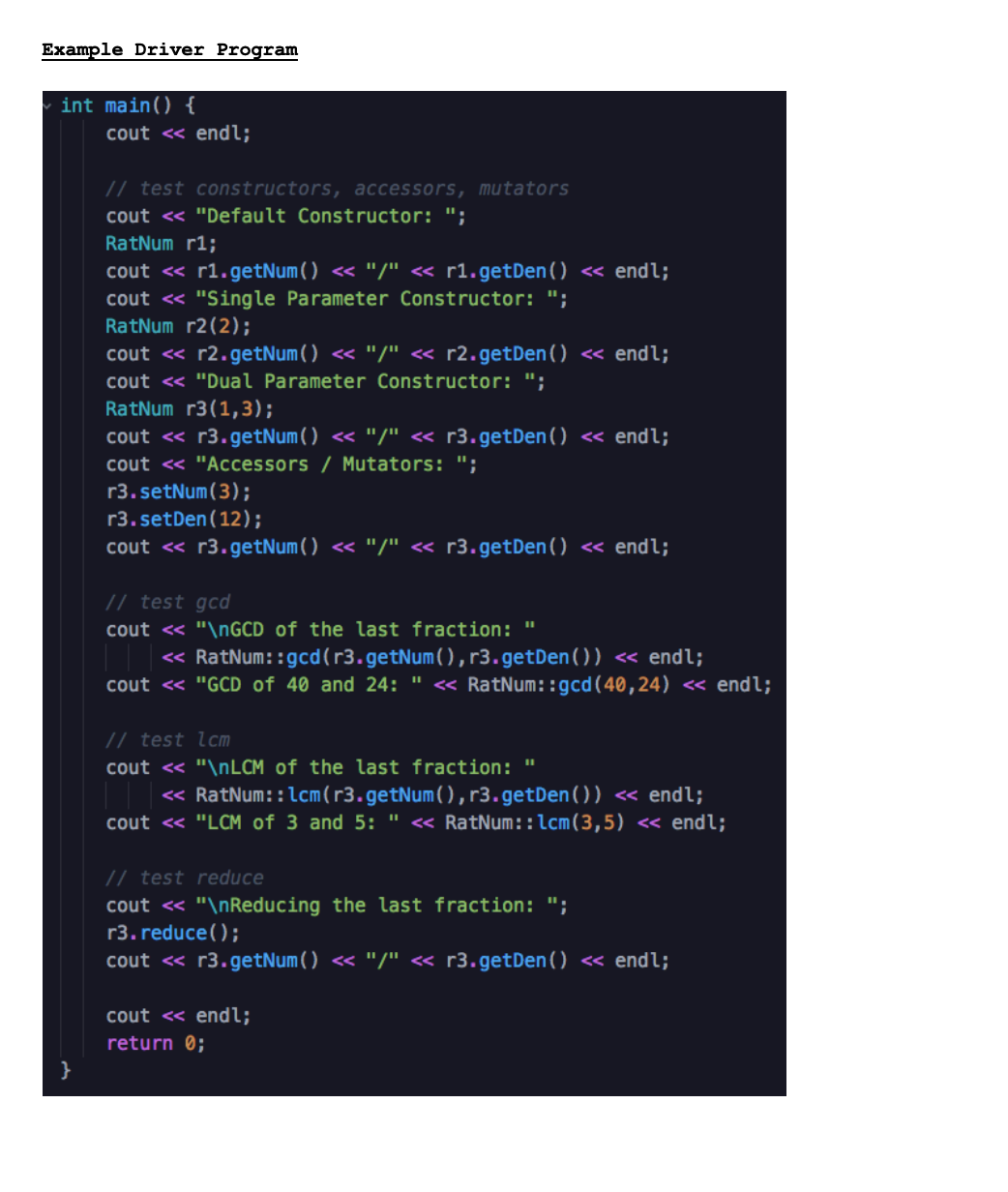help_outlineImage TranscriptioncloseExample Driver Program int main() { cout <« endl; // test constructors, accessors, mutators cout <« "Default Constructor: "; RatNum r1; cout « r1.getNum() <« "/" « r1.getDen() « endl; cout <« "Single Parameter Constructor: "; RatNum r2(2); cout « r2.getNum( ) << "/" « r2.getDen() « endl; cout <« "Dual Parameter Constructor: "; RatNum r3(1,3); cout <« r3.getNum() <« "/" « r3.getDen() « endl; cout <« "Accessors / Mutators: "; r3.setNum(3); r3.setDen(12); cout « r3.getNum( ) << "/" « r3.getDen() « endl; // test gcd cout <« "\NGCD of the last fraction: " « RatNum::gcd(r3.getNum(),r3.getDen()) <« endl; cout <« "GCD of 40 and 24: " « RatNum::gcd(40,24) « endl; // test lcm cout << "\NLCM of the last fraction: " « RatNum::lcm(r3.getNum(), r3.getDen()) « endl; cout <« "LCM of 3 and 5: " <« RatNum::lcm(3,5) <« endl; // test reduce cout << "\nReducing the last fraction: "; r3. reduce(); cout « r3.getNum( ) <« "/" « r3.getDen() « endl; cout « endl; return 0; fullscreen
check_circle

Step 1: Program

A C++ program for the given criteria is as follows,

File name: “main.cpp”

#include <iostream>

using namespace std;

class RatNum{

int numerator;

int denominator;

public:

//Default constructor

RatNum(){

numerator = 0; // numerator value 0

denominator = 1; //denominator value 1

}

//Single parameterised constructor

RatNum(int num){

numerator = num;

denominator = 1; //denominator value 1

}

//Dual parameterised constructor

RatNum(int num, int den){

numerator = num;

denominator = den;

}

//Set numerator

void setNum(int num){

numerator = num;

}

//Get numerator

int getNum(){

return numerator;

}

//Set denominator

void setDen(int den){

denominator = den;

}

//Get denominator

int getDen(){

return denominator;

}

//Find GCD

static int gcd(int a, int b){

if(a > b)

return gcd(a - b, b);

else if(a < b)

return gcd(a, b - a);

else

return a;

}

//Find lCM

static int lcm(int a, int b){

int ab = a * b;

int gcd = RatNum::gcd(a, b);

return ab / gcd;

}

//Reducing this fraction

void reduce(){

int gcd = RatNum::gcd(numerator, denominator);

numerator /= gcd;

denominator /= gcd;

}

};

int main(){

cout << endl;

//test constructor, accessor and mutator

cout << "Default Constructor: ";

RatNum r1;

cout << r1.getNum() << "/" << r1.getDen() << endl;

cout << "Single Parameter Constructor: ";

RatNum r2(2);

cout << r2.getNum() << "/" << r2.getDen() << endl;

cout << "Dual Parameter Constructor: ";

RatNum r3(1, 3);

cout << r3.getNum() << "/" << r3.getDen() << endl;

cout << "Accessors / Mutators: ";

r3.setNum(3);

r3.setDen(12);

cout << r3.getNum() << "/" << r3.getDen() << endl;

//test gcd

cout << "\nGCD of the last fraction: "

<< RatNum::gcd(r3.getNum(), r3.getDen()) << endl

<< "GCD of 40 and 24: " << RatNum::gcd(40, 24) << endl;

//test lcm

cout << "\nLCM of the last fraction: "

<< RatNum::lcm(r3.getNum(), r3.getDen()) << endl

<< "LCM of 3 and 5: " << RatNum::lcm(3, 5) << endl;

//test reduce

cout << "\nReducing last fraction: ";

r3.reduce();

cout << r3.getNum() << "/" << r3.getDen() << endl << endl;

return 0;

}

Step 2: Screenshot of program

Screenshot #1: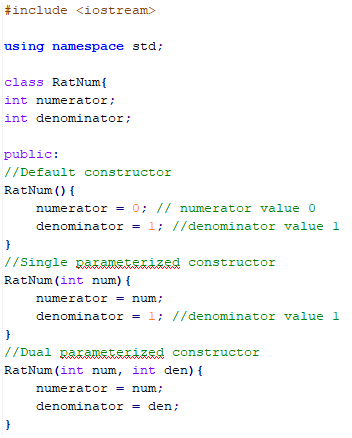Screenshot #2: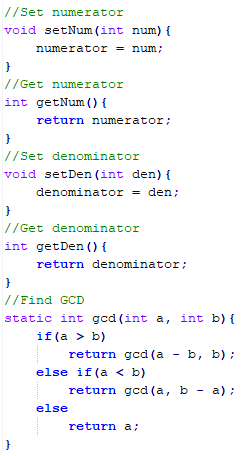Screenshot #3: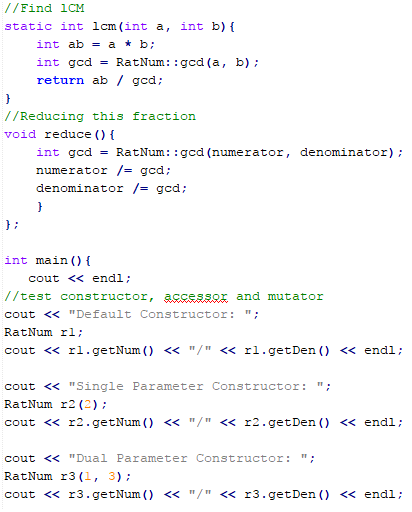Screenshot #4: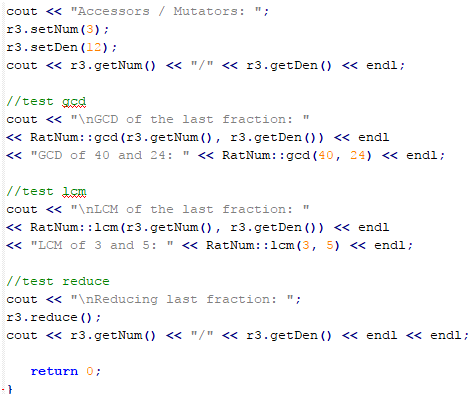...

### Want to see the full answer?

See Solution

#### Want to see this answer and more?

Solutions are written by subject experts who are available 24/7. Questions are typically answered within 1 hour.*

See Solution
*Response times may vary by subject and question.
Tagged in